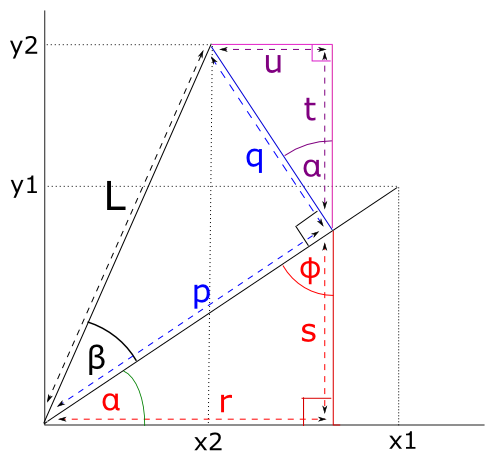$$\newcommand{L}{\| #1 \|}\newcommand{VL}{\L{ \vec{#1} }}\newcommand{R}{\operatorname{Re}\,(#1)}\newcommand{I}{\operatorname{Im}\, (#1)}$$

# Formula for rotating a vector in 2D¶

Let’s say we have a point $$(x_1, y_1)$$. The point also defines the vector $$(x_1, y_1)$$.

The vector $$(x_1, y_1)$$ has length $$L$$.

We rotate this vector anticlockwise around the origin by $$\beta$$ degrees.

The rotated vector has coordinates $$(x_2, y_2)$$

The rotated vector must also have length $$L$$.

## Theorem¶

$\begin{split}x_2 = \cos \beta x_1 - \sin \beta y_1 \\ y_2 = \sin \beta x_1 + \cos \beta y_1\end{split}$

## Preliminaries¶

Call the angle between $$(x_1, y_1)$$ and the x-axis : $$\alpha$$. Then:

(1)$\begin{split}x_1 = L \cos(\alpha) \\ y_1 = L \sin(\alpha)\end{split}$

We rotate $$(x_1, y_1)$$ by angle $$\beta$$ to get $$(x_2, y_2)$$. So the angle between $$(x_2, y_2)$$ and the x-axis is $$\alpha + \beta$$:

(2)$\begin{split}x_2 = L \cos(\alpha + \beta) \\ y_2 = L \sin(\alpha + \beta)\end{split}$

## Proof by the angle sum rule¶

If you are happy with The angle sum rule proof, then we are most of the way there.

The angle sum rule gives us:

$\begin{split}\cos(\alpha + \beta) = \cos \alpha \cos \beta - \sin \alpha \sin \beta \\ \sin(\alpha + \beta) = \sin \alpha \cos \beta + \cos \alpha \sin \beta\end{split}$

So, substituting from equations (1), (2):

$\begin{split}L \cos(\alpha + \beta) = L \cos \alpha \cos \beta - L \sin \alpha \sin \beta \implies \\ x_2 = x_1 \cos \beta - y_1 \sin \beta \\\end{split}$

We do the matching substitutions into $$\sin(\alpha + \beta)$$ to get $$y_2$$.

$$\blacksquare$$

## Proof by long-hand variant of angle sum proof¶

This section doesn’t assume the angle sum rule, but uses a version of the angle-sum proof to prove the rotation formulae.We can see from the picture that:

\begin{align}\begin{aligned}x_2 = r - u\\y_2 = t + s\end{aligned}\end{align}

We are going to use some basic trigonometry to get the lengths of $$r, u, t, s$$.

Because the angles in a triangle sum to 180 degrees, $$\phi$$ on the picture is $$90 - \alpha$$ and therefore the angle between lines $$q, t$$ is also $$\alpha$$.

Remembering the definitions of $$\cos$$ and $$\sin$$:

\begin{align}\begin{aligned}\cos\theta = \frac{A}{H} \implies A = \cos \theta H\\\sin\theta = \frac{O}{H} \implies O = \sin \theta H\end{aligned}\end{align}

Thus:

\begin{align}\begin{aligned}x_1 = \cos \alpha L\\y_1 = \sin \alpha L\\p = \cos \beta L\\q = \sin \beta L\\r = \cos \alpha p = \cos \alpha \cos \beta L = \cos \beta x_1\\s = \sin \alpha p = \sin \alpha \cos \beta L = \cos \beta y_1\\t = \cos \alpha q = \cos \alpha \sin \beta L = \sin \beta x_1\\u = \sin \alpha q = \sin \alpha \sin \beta L = \sin \beta y_1\end{aligned}\end{align}

So:

\begin{align}\begin{aligned}x_2 = r - u = \cos \beta x_1 - \sin \beta y_1\\y_2 = t + s = \sin \beta x_1 + \cos \beta y_1\end{aligned}\end{align}

$$\blacksquare$$.